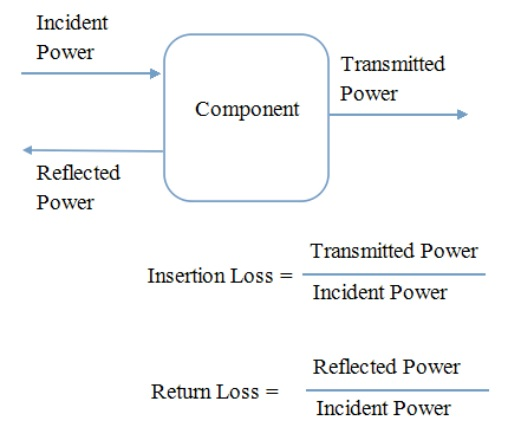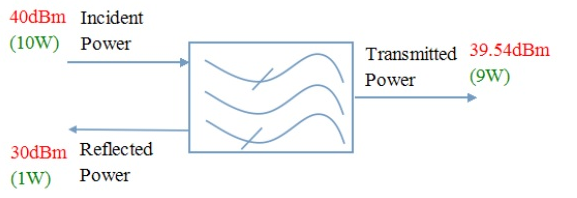# Insertion Loss / Return Loss

Insertion Loss, Return Loss and AttenuationIn order to understand losses, study the figure above.

Microwave power is sent down a transmission line from the left and it reaches the component. This power is the incident power. When it reaches the component, a portion is reflected back down the transmission line where it came from and never enters the component. Rest of the power gets into the component. There some of it gets absorbed and the remainder passes through the component into the transmission line on the other side. The power that actually comes out of the component is called the transmitted power, it is less than the incident power for two reasons: (1) some of the power gets reflected. (2) some of the power gets absorbed inside the component.

The ratio of incident power to transmitted power, in dB terminology, is the insertion loss. The ratio of incident power to the reflected power, in dB terminology, is the return loss.

Insertion Loss = 10 * Log (Incident power (W) / Transmitted power (W))

OR                    = Incident Power(dBm) – Transmitted Power(dBm)

Return Loss = 10 * Log (Incident(W) / Reflected power(W))

OR                 = Incident power (dBm) – Reflected power (dBm)

Example:• Insertion Loss = 40dBm – 39.54dBm  = 0.46dB
• Return Loss = 40dBm – 30dBm  = 10dB